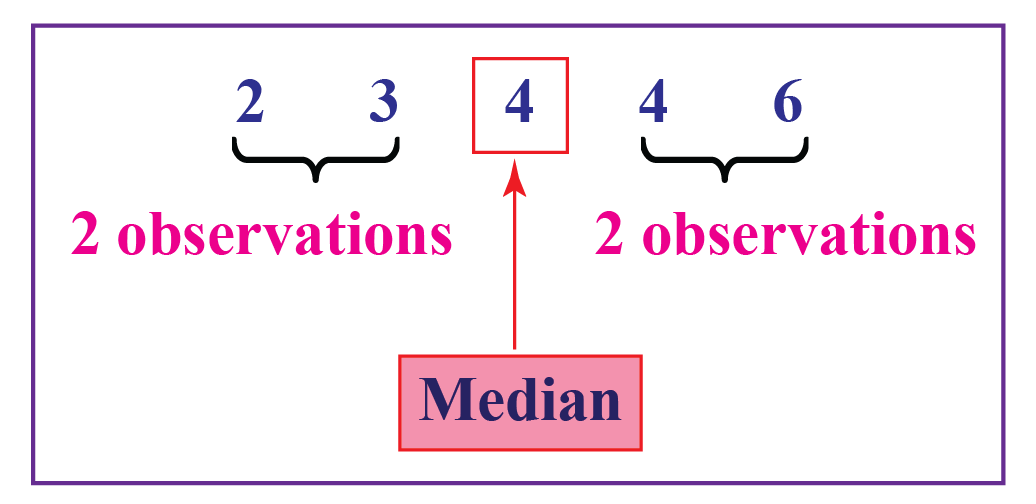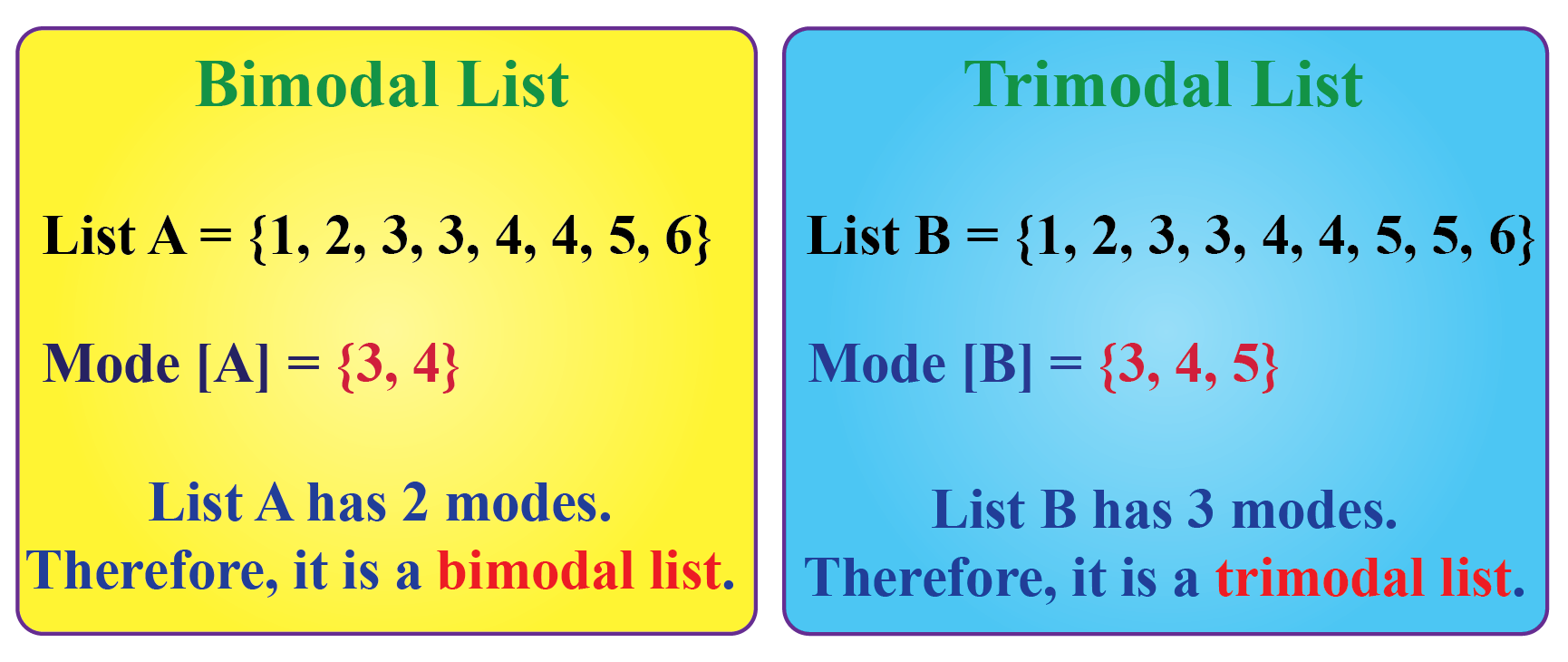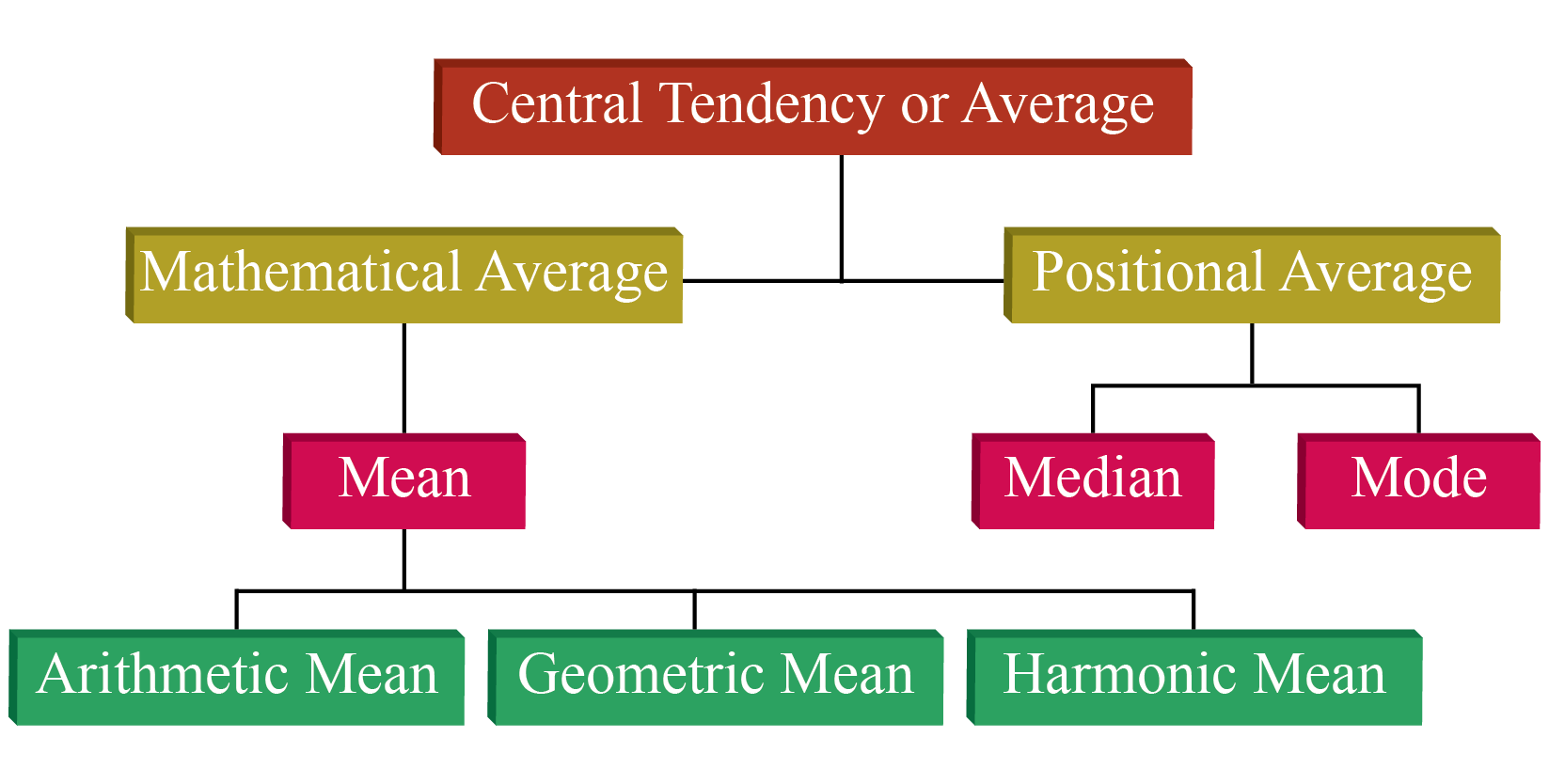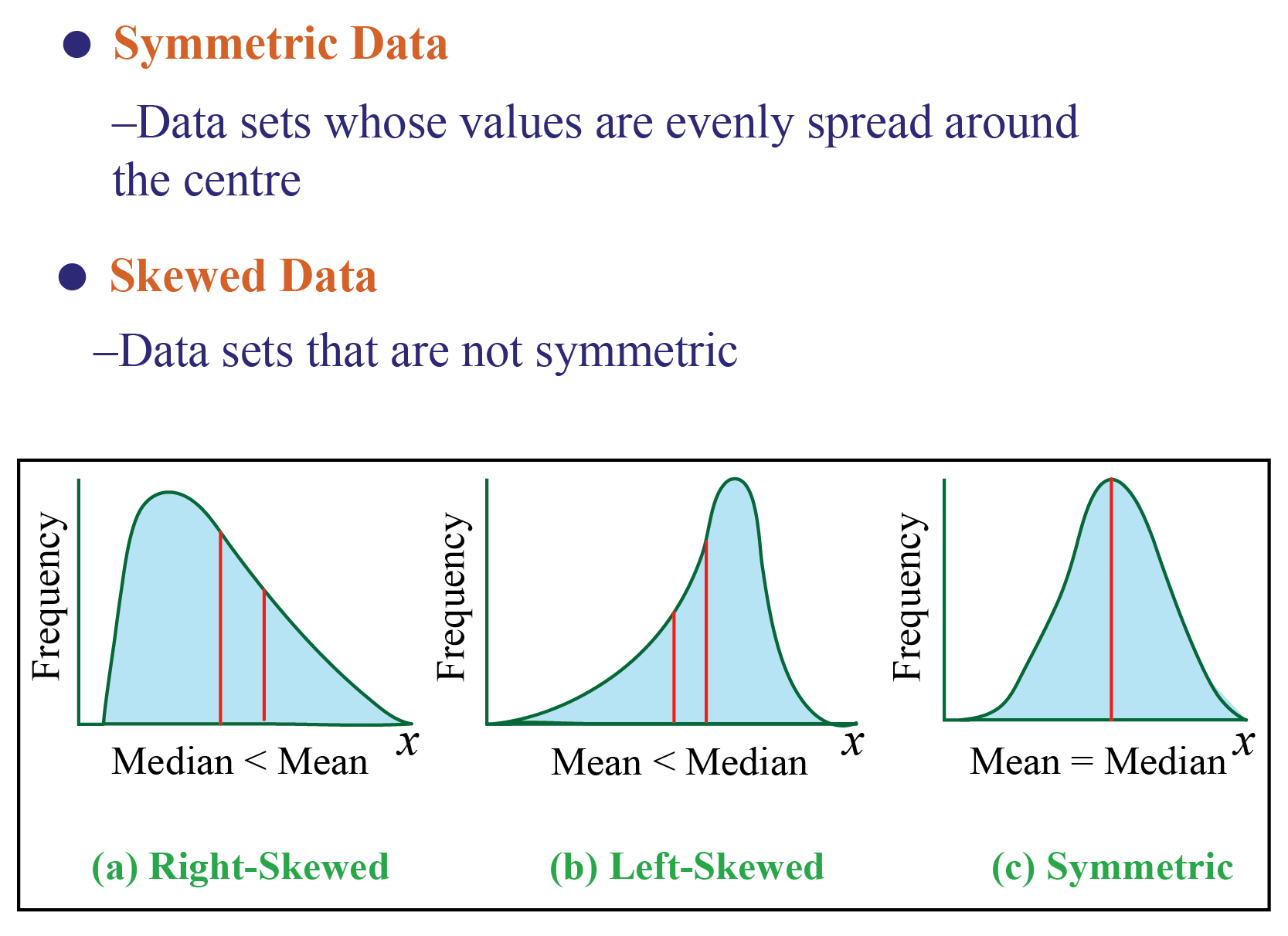# Mean, Median and Mode

Mean, Median and Mode

 1 Introduction to Mean, Median and Mode 2 Mean 3 Challenging Question 4 Median 5 Mode 6 Relation between Mean, Median, and Mode 7 Solved Examples on Mean, Median and Mode 8 Think Tank 9 Difference between Mean and Average 10 Difference between Mean and Median 11 Practice Questions 12 Maths Olympiad Sample Papers 13 Frequently Asked Questions (FAQs)

We at Cuemath believe that Math is a life skill. Our Math Experts focus on the “Why” behind the “What.” Students can explore from a huge range of interactive worksheets, visuals, simulations, practice tests, and more to understand a concept in depth.

Book a FREE trial class today! and experience Cuemath’s LIVE Online Class with your child.

## Introduction to Mean, Median And Mode

We come across data every day.

We find them in newspapers, articles, in our bank statements, mobile and electricity bills.

The list is endless; they are present all around us.

Now the question arises if we can figure out some important features of the data by considering only certain representatives of the data.

This is possible by using measures of central tendency or averages.

A measure of central tendency describes a set of data by identifying the central position in the data set as a single value.

We can think of it as a tendency of data to cluster around a middle value.

In statistics, the three most common measures of central tendencies are Mean, Median and Mode.

Choosing the best measure of central tendency depends on the type of data we have.

Let’s begin by understanding the meaning of each of these terms.

## Mean

The arithmetic mean of a given data is the sum of all observations divided by the number of observations.

For example: A cricketer's scores in five ODI matches are as follows: 12, 34, 45, 50, 24

To find his average score in a match, we calculate the arithmetic mean of data using the mean formula:

 $$\text {Mean = }\dfrac{\text {Sum of all observations}}{\text {Number of observations}}$$

\begin{align}\text{Mean } &=\dfrac{12+34+45+50+24}{5}\\\text{Mean } &=\dfrac{165}{5}\\ &=33\end{align}

Mean is denoted by $$\bar{x}$$ (pronounced as x bar)Challenging Questions

Let the mean of $$x_{1}$$, $$x_{2}$$, $$x_{3}$$ ……$$x_{n}$$  be A, then what is the mean of:

1. ($$x_{1}$$+k) ,($$x_{2}$$+k), ($$x_{3}$$+k),  ……($$x_{n}$$+k)?

2. ($$x_{1}$$-k) ,($$x_{2}$$-k), ($$x_{3}$$-k),  ……($$x_{n}$$-k)?

3. k$$x_{1}$$, k$$x_{2}$$, k$$x_{3}$$ ……k$$x_{n}$$?

### Types of Data

Data can be present in raw form or tabular form.

Let's find the mean in both cases.

### 1. Raw Data

Let $$x_{1}$$, $$x_{2}$$, $$x_{3}$$ ……$$x_{n}$$ be n observations.

We can find the arithmetic mean using the mean formula.

 $$\text {Mean,} \bar{x}=\dfrac{x_1+x_2+...x_n}{n}$$

Example 1

If the heights of 5 people are 142 cm, 150 cm, 149 cm, 156 cm, and 153 cm.

Find the mean height.

Mean height

\begin{align} &=\bar{x} \\ &=\dfrac{142+150+149+156+153}{5} \\ &=\dfrac{750}{5} \\ &=150 \end{align}

 Mean, $$\bar{x}$$ =150cm

Thus, the mean height is 150 cm.

### 2. Frequency Distribution (Tabular) Form

When the data is present in tabular form, we use the following formula:

 \begin{align}\text{Mean, }\bar{x}= \dfrac {x_1f_1 + x_2f_2+....x_nf_n}{\ f_+ f_2+.....f_n}\end{align}

Consider the following example.

Example 1

Find the mean of the following distribution:

 x 4 6 9 10 15 f 5 10 10 7 8

Solution

Calculation table for arithmetic mean:

 xi fi xifi 4 5 20 6 10 60 9 10 90 10 7 70 15 8 120 $$\sum f_i=40$$ $$\sum x_i f_i=360$$

\begin{align}
\therefore \text{Mean} &=\bar{x} \\
&=\dfrac{\sum x_if_i}{\sum f_i} \\
&=\dfrac{360}{40} \\
&=9
\end{align}

 Thus, Mean = 9

Example 2

Here is an example where the data is in the form of class intervals.

The following table indicates the data on the number of patients visiting a hospital in a month.

Find the average number of patients visiting the hospital in a day.

 Number of patients Number of days visiting hospital 0-10 2 10-20 6 20-30 9 30-40 7 40-50 4 50-60 2

Solution

In this case, we find the classmark (also called as mid-point of a class) for each class.

Note: $$\text {Class mark }=\dfrac{\text {lower limit + upper limit}}{2}$$

Let $$x_{1}$$, $$x_{2}$$, $$x_{3}$$ ……$$x_{n}$$ be the class marks of the respective classes.

Hence, we get the following table:

 Class mark (xi) frequency (fi) xifi 5 2 10 15 6 90 25 9 225 35 7 245 45 4 180 55 2 110 Total $$\sum f_i=30$$ $$\sum f_ix_i=860$$

\begin{align}
\therefore \text{Mean} =\bar{x} &=\dfrac{\sum x_if_i}{\sum f_i} \\
&=\frac{860}{30} \\
&=28.67
\end{align}

 $$\bar{x}=28.67$$

## Median

The value of the middlemost observation, obtained after arranging the data in ascending order, is called the median of the data.

For example, consider the data: 4, 4, 6, 3, 2.

Let's arrange this data in ascending order: 2, 3, 4, 4, 6.

There are 5 observations.

Thus, median = middle value i.e. 4

We can see here: 2, 3,  4, 4 , 6  (Thus, 4 is the median)### Case 1: Ungrouped Data

Step 1: Arrange the data in ascending or descending order.

Step 2: Let the total number of observations be n.

To find the median, we need to consider if n is even or odd.

If $$n$$ is odd, then use the formula:

 $$\text { Median = } (\dfrac {n+1}{2})^{th }\text {observation}$$

Example 1

Let's consider the data: 56, 67, 54, 34, 78, 43, 23.

What is the median?

Solution

Arranging in ascending order, we get: 23, 34, 43, 54, 56, 67, 78.

Here, n (no.of observations) = 7

So,

\begin{align}  \dfrac{7+1}{2}&=4 \\\therefore Median &= 4^{th}\text { observation}\end{align}

 Median = 54

If $$n$$ is even, then use the formula:

 $$\text { Median = } \dfrac {\dfrac{n}{2}^{th}\text {obs.}+ (\dfrac{n}{2}+1)^{th}\text {obs.}}{2}$$

Example 2

Let's consider the data: 50, 67, 24, 34, 78, 43.

What is the median?

Solution

Arranging in ascending order, we get: 24, 34, 43, 50, 67, 78.

Here, n (no.of observations) = 6

$$\dfrac{6}{2}=3$$

Using the median formula,

\begin{align} \text { Median } &=\dfrac {3^{rd}\text {obs.}+ 4^{th}\text {obs.}}{2} \\&=\dfrac{43+50}{2}\end{align}

 Median = 46.5

### Case 2: Grouped Data

When the data is continuous and in the form of a frequency distribution, the median is found as shown below:

Step 1: Find the median class.

Let n = total number of observations i.e. $$\sum f_i$$

Note: Median Class is the class where $$\dfrac{n}{2}$$ lies.

Step 2: Use the following formula to find the median.

 $$\text { Median = } l + [\dfrac {\dfrac{n}{2}-c}{f}]\times h$$

where,

$$l=$$ lower limit of median class

$$c=$$ cumulative frequency of the class preceding the median class

$$f=$$ frequency of the median class

$$h=$$ class size

Let's consider the following example to understand this better.

Example 1

Find the median marks for the following distribution:

 Classes 0-10 10-20 20-30 30-40 40-50 Frequency 2 12 22 8 6

Solution

We need to calculate the cumulative frequencies to find the median.

Calculation table:

 Classes Number of students Cumulative frequency 0-10 2 2 10-20 12 2 + 12 = 14 20-30 22 14 + 22 = 36 30-40 8 36 + 8 = 44 40-50 6 44 + 6 = 50

$$N=50$$

$$\dfrac{N}{2} = \dfrac{50}{2}= 25$$

Median Class $$= 20-30$$

$$l = 20, f =22, c.f= 14, h =10$$

Using Median formula:

\begin{align}\text { Median  } &=l + [\dfrac {\dfrac{n}{2}-c}{f}]\times h\\&=20 + \dfrac{25-14}{22} \times 10 \\&= 20 + \dfrac{11}{22} \times 10 \\&=20+5= 25 \end{align}

 $$\therefore$$  Median = 25

## Mode

The value which appears most often in the given data i.e. the observation with the highest frequency is called a mode of data.

### Case 1: Ungrouped Data

For ungrouped data, we just need to identify the observation which occurs maximum times.

Mode = Observation with maximum frequency

For example in the data: 6, 8, 9, 3, 4, 6, 7, 6, 3 the value 6 appears the most number of times.

Thus, mode = 6.

An easy way to remember mode is: Most Often Data Entered.

Note: A data may have no mode, 1 mode, or more than 1 mode.

Depending upon the number of modes the data has, it can be called unimodal, bimodal, trimodal, or multimodal.

The example discussed above has only 1 mode, so it is unimodal.### Case 2: Grouped Data

When the data is continuous, the mode can be found using the following steps:

Step 1: Find modal class i.e. the class with maximum frequency.

Step 2: Find mode using the following formula:

 $$\text { Mode = } l + [\dfrac {f_m-f_1}{2f_m-f_1-f_2}]\times h$$

where, $$l=$$ lower limit of modal class,

$$f_m =$$ frequency of modal class,

$$f_1=$$ frequency of class preceding modal class,

$$f_2=$$ frequency of class succeeding modal class,

$$h=$$ class width

Consider the following example to understand the formula.

Example 1

Find the mode of the given data:

 Marks Obtained 0-20 20-40 40-60 60-80 80-100 Number of students 5 10 12 6 3

Solution

The highest frequency $$=$$ 12, so the modal class is 40-60.

$$l=$$ lower limit of modal class = 40

$$f_m =$$ frequency of modal class =12

$$f_1=$$frequency of class preceding modal class = 10

$$f_2=$$frequency of class succeeding modal class = 6

$$h=$$class width $$=$$ 20

Using the mode formula,

\begin{align} \text { Mode } &= l + [\dfrac {f_m-f_1}{2f_m-f_1-f_2}]\times h\\&= 40+[\dfrac{12-10}{2 \times 12 - 10-6} ]\times 20\\&= 40+[\dfrac{2}{8} ]\times 20\\&= 45 \end{align}

 $$\therefore$$ Mode = 45

Let's try finding mean, median, and mode in the case mentioned below.

Instructions: Click on the green board and observe the mean, median, and mode of the numbers appearing on the screen.

Verify the answer by calculating using the formula discussed above.

Help your child score higher with Cuemath’s proprietary FREE Diagnostic Test. Get access to detailed reports, customized learning plans, and a FREE counseling session. Attempt the test now.

## Relation Between Mean, Median and Mode

The three measures of central values i.e. mean, median, and mode are closely connected by the following relations (called an empirical relationship).

$$\text {2Mean + Mode =3Median}$$

For instance, if we are asked to calculate the mean, median, and mode of continuous grouped data, then we can calculate mean and median using the formulae as discussed in the previous sections and then find mode using the empirical relation.

For example, we have a data whose mode $$=$$ 65 and median $$=$$ 61.6.

Then, we can find the mean using the above relation.

\begin{align}
2 \text {Mean}+ \text {Mode} &=3 \text { Median } \\
\therefore 2  \operatorname{Mean} &=3 \times 61.6-65 \\
\therefore 2 \operatorname{Mean} &=119.8 \\
\Rightarrow \text {Mean} &=\dfrac{119.8}{2} \\\Rightarrow \text {Mean} &= 59.9
\end{align}

## Solved Examples on Mean, Median and Mode

 Example 1

If the mean of the following data is 20.6, find the missing frequency (p).

 x 10 15 20 25 35 f 3 10 p 7 5

Solution:

Let us make the calculation table for this :

 xi fi xifi 10 3 10 x 3 = 30 15 10 15 x 10 = 150 20 p 20 x p = 20p 25 7 25 x 7= 175 35 5 35 x 5 = 175 Total: $$\sum {f_i}=25+p$$ $$\sum {f_ix_i}$$ = $$530+20p$$

\begin{align}
\text {Mean} &=\dfrac{\sum f_ix_i}{\sum f_i} \\
20.6&= \dfrac{530+ 20p}{25+p} \\
530 + 20p&=515+20.6p \\15 &= 0.6p \\p &=\dfrac{15}{0.6}\\&=25
\end{align}

 $$\therefore$$ The missing frequency (p) =25
 Example 2

The mean of 5 numbers is 18

If one number is excluded, their mean is 16

Find the excluded number.

Solution:

Given, n = 5, $$\bar{x}$$ = 18

$$\therefore$$ $$\bar{x}$$ = $$\dfrac{\sum {x_i}}{n}$$

$$\sum {x_i}$$= $$5\times 18$$ = 90

Thus, the total of 5 numbers = 90

Let the excluded number be $$a$$.

Therefore, total of 4 numbers$$=90-a$$

\begin{align} \text {Mean of 4 numbers} &=\dfrac{90-a}{4} \\ 16&= \dfrac{90-a}{4} \\ 90-a&= 64 \\ \end{align}

 $$\therefore \text{a} = 26$$
 Example 3

A survey on the heights (in cm) of 50 girls of class X was conducted at a school and the following data was obtained:

 Height (in cm) Number of girls 120-130 130-140 140-150 150-160 160-170 Total 2 8 12 20 8 50

Find the mode and median of the above data.

Solution:

\begin{align} \text {Modal class} &= 150-160\end{align}

[as it has maximum frequency]

\begin{align}l =150, h &=10, f_m =20, f_1 =12,f_2=8 \\ Mode&= l +\dfrac{f_m-f_1}{2f_m-f_1-f_2}\times h \\ &= 150 +\dfrac{20-12}{2 \times 20-12-8}\times 10\\ &= 150 +4\\&=154\\ \therefore \text {Mode}&=154 \end{align}

To find the median, we need cumulative frequencies.

Consider the table:

 Class Intervals No. of girls (fi) Cumulative frequency (c) 120-130 2 2 130-140 8 2+8=10 140-150 12 = f1 10+12=22 (c) 150-160 20 = fm 22+20=42 160-170 8 = f2 42+8=50 (n)

\begin{align} n &= 50 \\ \dfrac{n}{2} &=25 \\\end{align}

\begin{align} \therefore\text {Median class} &= 150-160\\
l =150, c= 22, f&=20, h= 10\\ \text {Median }&= l +\dfrac{\dfrac{n}{2}- c}{f} \times h \\
&= 150+\dfrac{\dfrac{50}{2}- 22}{20} \times 10 \\ &= 150+ 1.5\\&=151.5
\end{align}

 $$\therefore$$ Mode = 154, Median= 151.5Think Tank

There were five questions, each carrying ten marks.

Their scores were as follows:

 Question Number 1 2 3 4 5 Heena's score 10 8 9 8 7 Harry's score 4 7 10 10 10

Both of them got into an argument.

Heena said that she had a better average score so she was a better performer.

Whereas Harry argued that he had scored 10 out of 10 most number of times so he was a better performer.

Help them decide who performed better.

## Difference between Mean and Average

The term average is frequently used in everyday life to denote a value that is typical for a group of quantities.

Average rainfall in a month or the average age of employees of an organization is typical examples.

We might read an article stating "People spend an average of 2 hours every day on social media. "

We understand from the use of the term average that not everyone is spending 2 hours a day on social media but some spend more time and some less.

However, we can understand from the term average that 2 hours is a good indicator of the amount of time spent on social media per day.

Most people use average and mean interchangeably even though they are not the same.

• Average is the value that indicates what is most likely to be expected.
• They help to summarise large data into a single value.

An average tends to lie centrally with the values of the observations arranged in ascending order of magnitude.

So, we call an average measure of the central tendency of the data.

Averages are of different types.

What we refer to as mean i.e. the arithmetic mean is one of the averages.

Mean is called the mathematical average whereas median and mode are positional averages.## Difference between Mean and Median

Mean is known as the mathematical average whereas the median is known as the positional average.

To understand the difference between the two, consider the following example.

A department of an organization has 5 employees which include a supervisor and four executives.

The executives draw a salary of ₹10,000 per month while the supervisor gets ₹40,000.

Mean,

\begin{align} &=\dfrac{10000+10000+10000+10000+40000}{5}\\&=\dfrac{80000}{5}=16000\end{align}

Thus, the mean salary is ₹16,000.

To find the median, we consider the ascending order: 10000, 10000, 10000, 10000,  40000.

$$n=5, so\dfrac{n+1}{2}=3$$ .

Thus, the median is the 3rd observation.

$$Median = 10000$$

Thus, the median is ₹10,000 per month.

Now let us compare the two measures of central tendencies.

We can observe that the mean salary of ₹16,000 does not give even an estimated salary of any of the employees whereas the median salary represents the data more effectively.

One of the weaknesses of mean is that it gets affected by extreme values.

Look at the following graph to understand how extreme values affect mean and median:So, mean is to be used when we don't have extremes in the data.

If we have extreme points, then the median gives a better estimation.

Here's a quick summary of the differences between the two.

Mean Vs Median
Mean Median
Definition Average of given data (Mathematical Average) Central value of data (Positional Average)
Calculation Add all values and divide by total number of observations Arrange data in ascending / descending order and find middle value
Values of data Every value is considered for calculation Every value is not considered
Effect of extreme points  Greatly effected by extreme points Doesn't get effected by extreme points

## Practice Questions

Here are a few activities for you to practice. Select/Type your answer and click the "Check Answer" button to see the result.

IMO (International Maths Olympiad) is a competitive exam in Mathematics conducted annually for school students. It encourages children to develop their math solving skills from a competition perspective.

## Q1. What is the mode value?

It is the value that appears maximum number of times in data.

It is best to put the numbers in order and then, count the number of times each value occurs.

The value with the highest frequency will be the modal value of the data.

## Q2. What does mode tell you?

A mode represents the most common value.

It tells you which value has occurred most often in the given data.

On a bar chart, the mode is the highest bar.

It is used with categorial data such as most sold T-shirts size.

## Q3. How do I calculate the median?

Median is the value of the middlemost observation, obtained after arranging the data in ascending order.

To find the same, we need to consider two cases.

$$\text {Median=} (\dfrac {n+1}{2})^{th }\text { observation}$$ , $$\text {when n is odd }$$

$$\text {Median=} \dfrac {\dfrac{n}{2}^{th}\text {obs.}+ (\dfrac{n}{2}+1)^{th}\text {obs.}}{2}$$ , $$\text {when n is even }$$

For grouped data, the median is obtained using the median formula:

$$\text {Median= } l + [\dfrac {\dfrac{n}{2}-c}{f}]\times h$$

## Q4. Are mean and median the same?

No, mean and median are not the same.

• Mean is the average of the given sets of numbers. We need to add the numbers up then divide their sum by the number of observations.
• For finding the mode, we find whether any number appears more than once. The number which appears most is the mode.

• If there are other numbers that repeat to the same level, there may be more than one mode. A set could be bimodal or trimodal. But the mean of a given data is unique.
More Important Topics
Numbers
Algebra
Geometry
Measurement
Money
Data
Trigonometry
Calculus